# ?uestion 3: Settling Tank Performance A rectangular primary settling tank in a wastewater treatment plant is...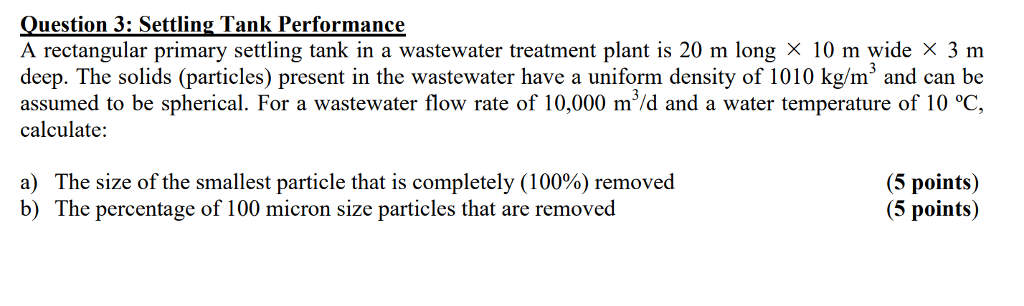?uestion 3: Settling Tank Performance A rectangular primary settling tank in a wastewater treatment plant is 20 m long × 10 m wide x 3 m deep. The solids (particles) present in the wastewater have a uniform density of 1010 kg/m3 and can be assumed to be spherical. For a wastewater flow rate of 10,000 m'/d and a water temperature of 10 °C, calculate: a) The size of the smallest particle that is completely (100%) removed b) The percentage of 100 micron size particles that are removed (5 points) (5 points)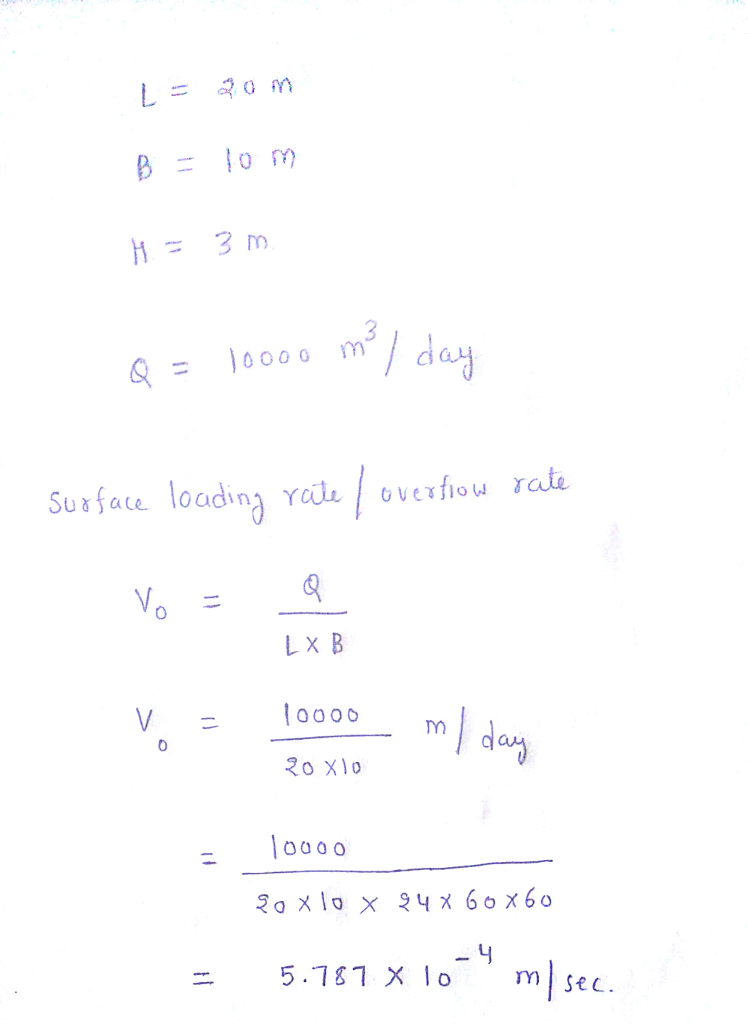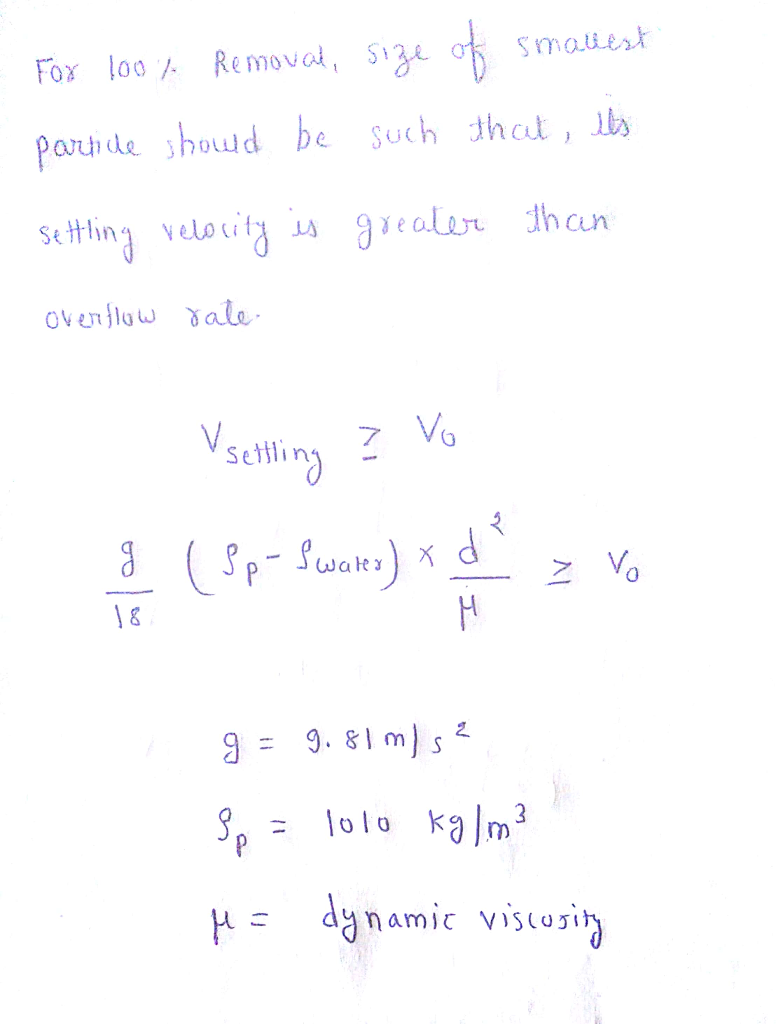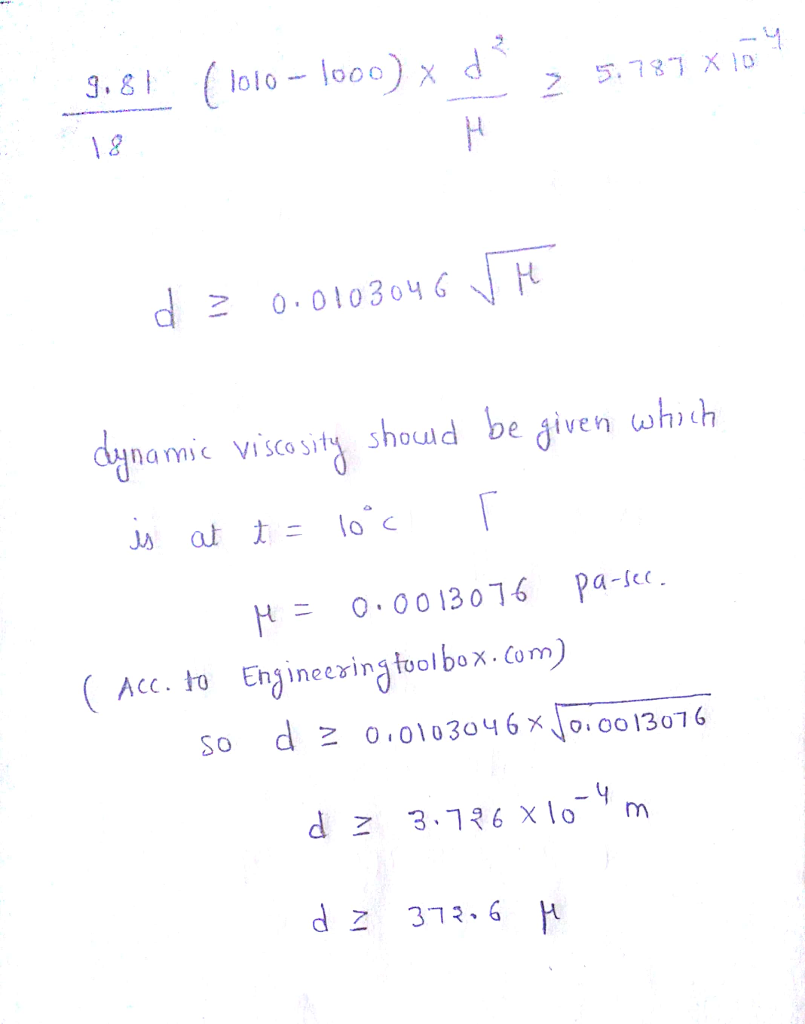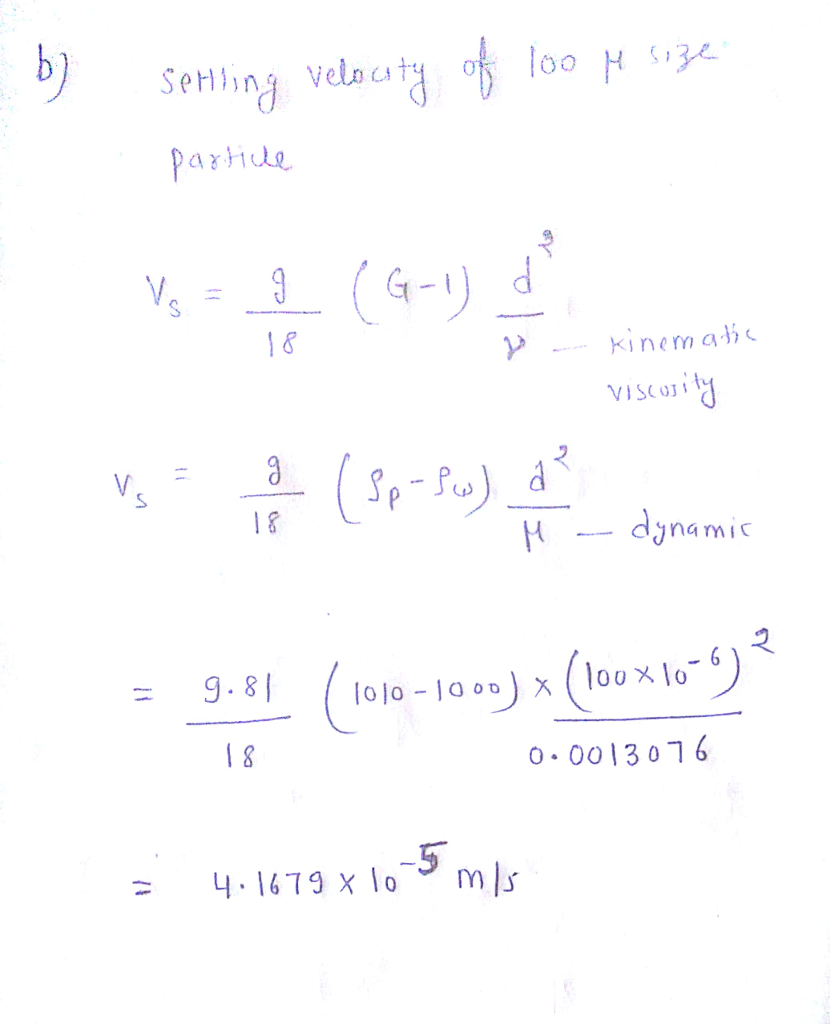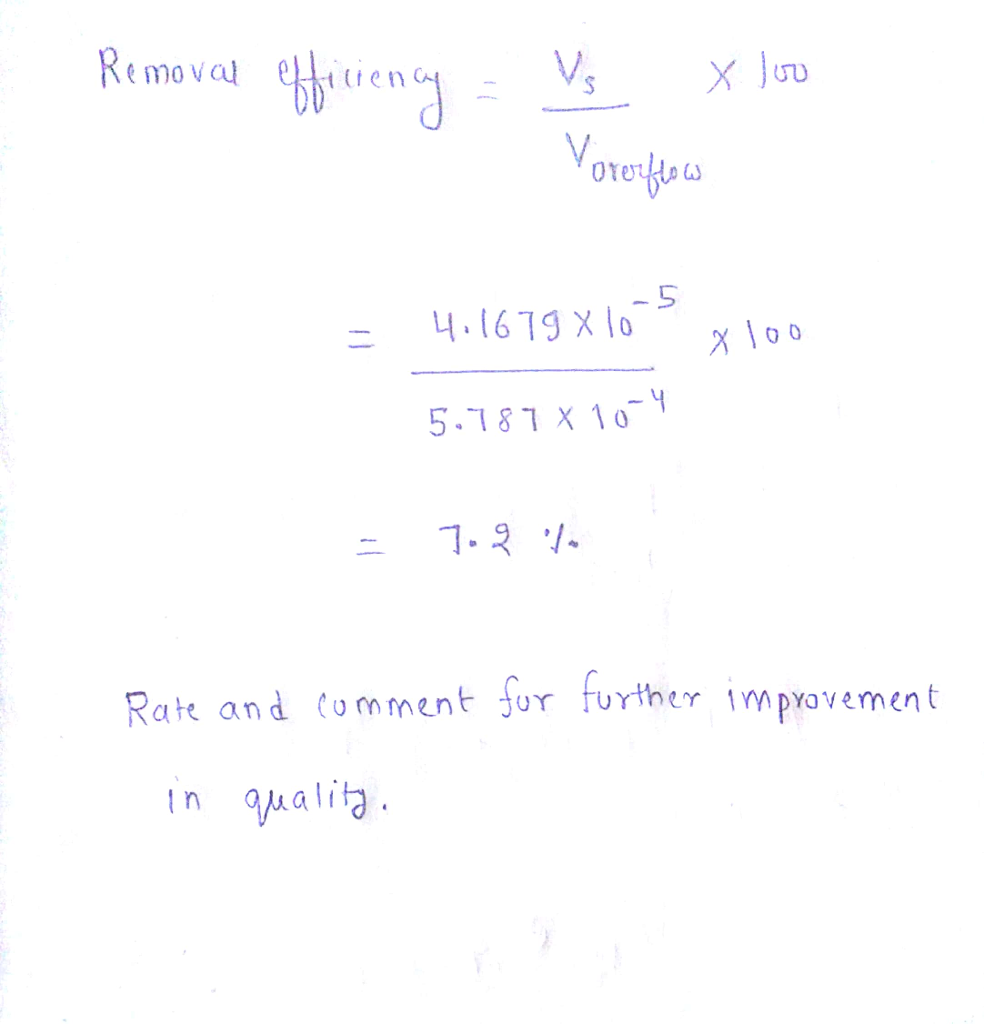#### Earn Coin

Coins can be redeemed for fabulous gifts.

Similar Homework Help Questions
• ### You are hired to design a rectangular sedimentation tank (length to width ratio = 3:1 and...

You are hired to design a rectangular sedimentation tank (length to width ratio = 3:1 and detention time ~ 2 h) for a new water treatment plant (flow rate: 0.04 m3/s; temperature: 20 ℃). Suspended particles can be removed when the overflow rate is higher than the settling velocities of particles. You have finished characterizing the influent particles (assuming all of them are spherical) and obtained the following results: Particle diameter (m) Particle density (kg/m3) Percentile 50 1.4 x 103...

• ### You are hired to design a rectangular sedimentation tank (length to width ratio = 3:1 and...

You are hired to design a rectangular sedimentation tank (length to width ratio = 3:1 and detention time ~ 2 h) for a new water treatment plant (flow rate: 0.04 m3/s; temperature: 20 ℃). Suspended particles can be removed when the overflow rate is higher than the settling velocities of particles. You have finished characterizing the influent particles (assuming all of them are spherical) and obtained the following results: Particle diameter (μm) Particle density (kg/m3) Percentile ≥ 50 ≥ 1.4...

• ### You are hired to design a rectangular sedimentation tank (length to width ratio = 3:1 and...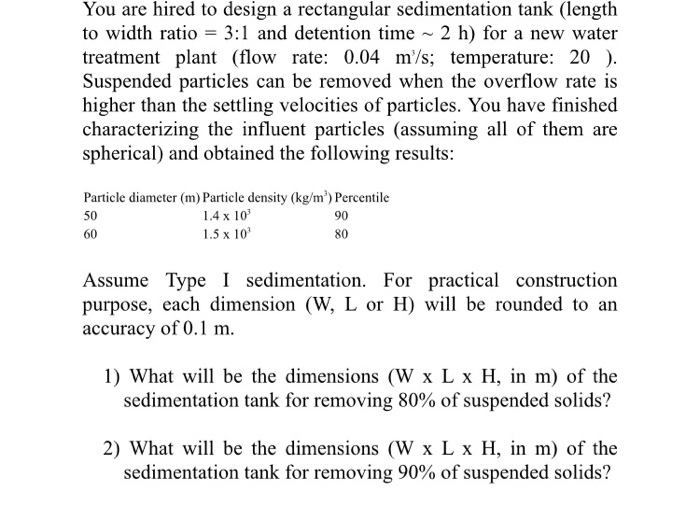You are hired to design a rectangular sedimentation tank (length to width ratio = 3:1 and detention time ~ 2 h) for a new water treatment plant (flow rate: 0.04 m/s; temperature: 20 ). Suspended particles can be removed when the overflow rate is higher than the settling velocities of particles. You have finished characterizing the influent particles (assuming all of them are spherical) and obtained the following results: Particle diameter (m) Particle density (kg/m) Percentile 50 1.4 x 10...

• ### Problem 2 (20 points) You are hired to design a rectangular sedimentation tank (length to width...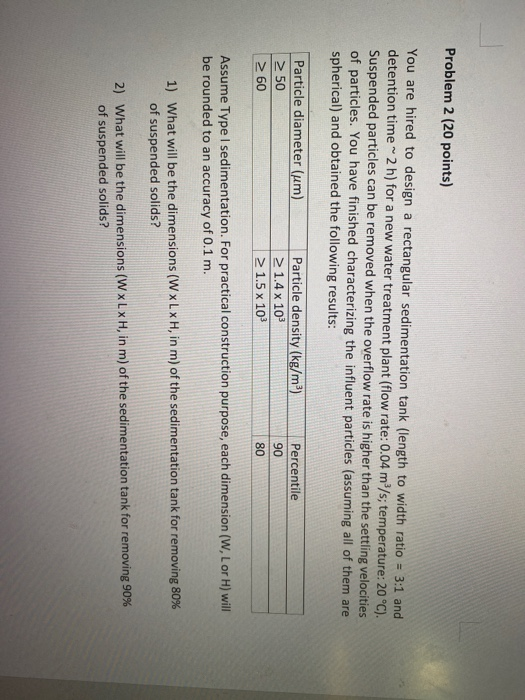Problem 2 (20 points) You are hired to design a rectangular sedimentation tank (length to width ratio = 3:1 and detention time 2 h) for a new water treatment plant (flow rate: 0.04 m/s; temperature: 20 °C). Suspended particles can be removed when the overflow rate is higher than the settling velocities of particles. You have finished characterizing the influent particles (assuming all of them are spherical) and obtained the following results: Percentile Particle diameter (um) > 50 > 60...

• ### 2.  A small town has been directed to upgrade its primary wastewater treatment plant to...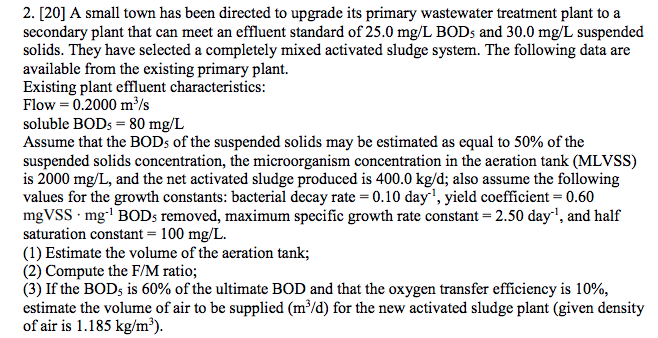2.  A small town has been directed to upgrade its primary wastewater treatment plant to a secondary plant that can meet an effluent standard of 25.0 mg/L BODs and 30.0 mg/L suspended solids. They have selected a completely mixed activated sludge system. The following data are available from the existing primary plant. Existing plant effluent characteristics Flow = 0.2000 m 3/s soluble BODs-80 mg/L Assume that the BOD5 of the suspended solids may be estimated as equal to 50%...

• ### 02: A rectangular settling tank with a width of (6 m), flow of raw water is...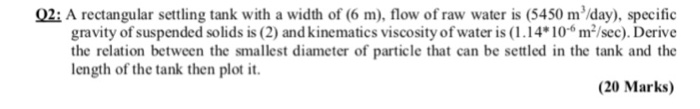02: A rectangular settling tank with a width of (6 m), flow of raw water is (5450 m /day), specific gravity of suspended solids is (2) and kinematics viscosity ofwater is (1.14 106m/sec). Derive the relation between the smallest diameter of particle that can be settled in the tank and the length of the tank then plot it (20 Marks)

• ### Primary clarifier is to be designed for a municipal wastewater treatment plant with a flow of 416...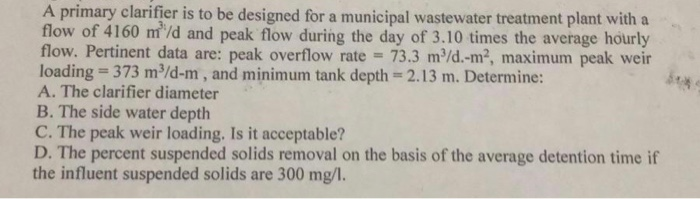primary clarifier is to be designed for a municipal wastewater treatment plant with a flow of 4160 m/d and peak flow during the day of 3.10 times the average hourly flow. Pertinent data are: peak overflow rate 73.3 m/d.-m2, maximum peak weir loading 373 m3/d-m, and minimum tank depth 2.13 m. Determine: A. The clarifier diameter B. The side water deptlh C. The peak weir loading. Is it acceptable? D. The percent suspended solids removal on the basis of the...

• ### :A wastewater treatment plant consisted of a primary sedimentation tank and two stages of trickling filter...

:A wastewater treatment plant consisted of a primary sedimentation tank and two stages of trickling filter received flow 44L/Sec , the BOD concentration in raw sewage =440 mg/L , 30% BOD removed in the primary sedimentation tank, volume of filter (first stage )=826000L, volume of filter (second stage)=2563000L, recirculation rate =0.5 in second tank while no recirculation in first tank. Determine the following: 1-The effluent concentration of BOD in each unit. 2-Treatment efficiency of each unit. 3-Treatment efficiency of plant....

• ### Diameter must equal .25 cm or please don’t answer! The specific gravity of the particles being...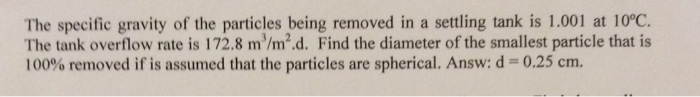Diameter must equal .25 cm or please don’t answer! The specific gravity of the particles being removed in a settling tank is 1.001 at 10°C. The tank overflow rate is 172.8 m/m2.d. Find the diameter of the smallest particle that is 100% removed if is assumed that the particles are spherical. Answ: d-: 0.25 cm.

• ### Question #2 (25 points) A water treatment facility has 4 primary sedimentation tanks that operate in...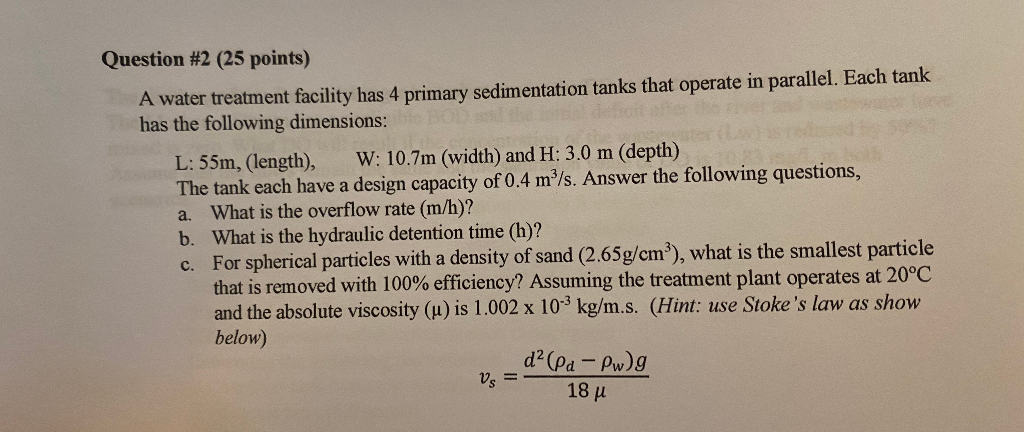Question #2 (25 points) A water treatment facility has 4 primary sedimentation tanks that operate in parallel. Each tank has the following dimensions: L: 55m, (length), W: 10.7m (width) and H: 3.0 m (depth) The tank each have a design capacity of 0.4 m”/s. Answer the following questions, a. What is the overflow rate (m/h)? b. What is the hydraulic detention time (h)? c. For spherical particles with a density of sand (2.65g/cm²), what is the smallest particle that is...